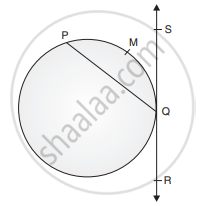# In the following figure, m(arc PMQ) = 130o, find ∠PQS. - Geometry

Sum

In the following figure, m(arc PMQ) = 130o, find ∠PQS.#### SolutionGiven : m(arc PMQ) = 130°

According to Tangent Chord Theorem,
The tangent chord angle theorem states that the measure of the angle formed by the intersection of a tangent and a chord of the circle equals one half of the intercepted arc by the chord.

∴ ∠PQS = 1/2 m(arc PMQ)

∠PQS = [130°]/2 = 65°

Concept: Angle Subtended by the Arc to the Point on the Circle
Is there an error in this question or solution?